# Steam and Gas Turbines - The Rankine Cycle

Al_Pa_Cone
<<Moved from another forum, thus no template filled out.>>

I am having some trouble trying to relate a question given in my distance learning lessons to the equivelent question in my assignment.

The assignement question is:

1(a) Superheated steam at a pressure of 40 bar and a temperature of 500°C is supplied to the turbine of a Rankine cycle. If the condenser pressure is 0.03 bar, find the thermal efficiency of the cycle. Neglect feed pump work.

I am instructed to use steam tables from:

"Thermodynamic and Transport Properties of Fluids"

S I Units
arranged by
G F C Rogers and Y R Mayhew
Basil Blackwell

Which I found a free online version of here:
https://www.slideshare.net/SGhallab/steam-tables-fifth-edition-by-rogers-and-mayhew

The Rankine Cycle I understand can be divided into two halfs, High pressure (Steam entering the turbine) and Low Pressure (Steam entering the condenser).

The Symbol notation in the below chart is as follows: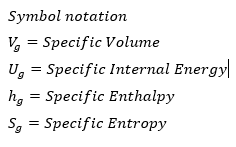From the question superheated steam at a pressure of 40 bar and a temperature of 500°C would fall into this highlighted catagorie of the steam tables, and I am looking at the Enthalpy (the amount of internal energy required to conver 1kg of water to 1kg of steam) so I get 3445 kJ kg^-1

In my notes I have the the Thermal Efficiency (TE) = (h_1 -h_2)/(h_1-h_3)
h_1 i believe is 3445 kj kg^-1
h_2 is the Specific Enthalpy after the steam leaves the turbine and enters the condenser, so I am looking at the 0.03 bar pressure at 500°C and I understand I should use Interpolation to find the figure at 0.03 as the given figures are 0.01 to 0.05.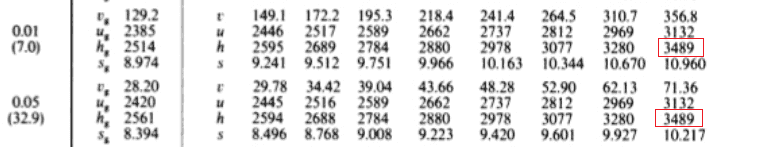but I can see it is unchanged from the highlighted 3489 kJ kg^-1

Now I have a problem! In the lessons the example used gives a totally different answer to obtain h_2 and I cannot figure out how they got it.

Can anyone verify I am on the right course so far?

Thank you!

#### Attachments

Last edited by a moderator:

Mentor
The entropy change is supposed to be zero in the turbine.

Al_Pa_Cone
I am told the procces has very little heat loss through the turbine therefore the temperature is constant. When the superheated steam enters the low pressure condensor from my chart I can see the steam must be cooled to 19.95deg which is half way between (7.0) and (32.9) at pressures 0.01 to 0.05 which should be the figure for 0.03bar.

I can see the entropy at 19.95deg would be 8.684 (half way between the given 8.974 and 8.394) and the entropy at 500deg upon entering the condenser would be between 10.217 and 10.960 so the mid point would be 10.535 kJ kg^-1 K-1.
So from this the change in entropy is 10.535 - 8.684 = 1.851 or is it?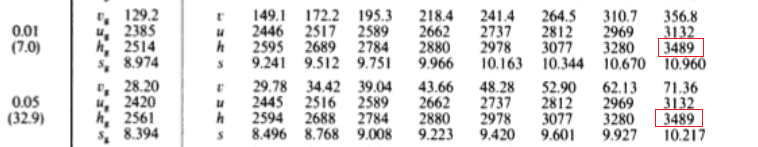I am confused because if I go to the extract in the steam tables at approximately 20deg (19.95deg from the above solution)

Temp Pressure Volume Specific Enthalpy SE Steam(hg) S Entropy S Entropy
Water(hf) Water(Sf) Steam(Sg)
SE Evaporation(hfg) SEntropy Evap(Sfg)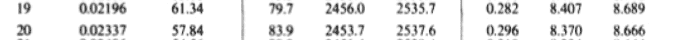I Hope the above headings make sense...

My entropy method looks correct at 8.684 but the change in entropy is different. Now the superheated steam is now cooled am I dealing with Enthalpy (hfg)?

I can't picture what is going on and I feel like I am going round in circles with the lessons provided.

#### Attachments

Just a quick literature tip: "An introduction to thermal physics" by Schroeder has a simple and instructive treatment of the steam turbine.

Mentor
I am told the procces has very little heat loss through the turbine therefore the temperature is constant.
This is definitely NOT CORRECT. The turbine is doing work, so there is a huge decrease in enthalpy (at constant entropy, as I said), and a corresponding large decrease in temperature. The temperature exiting the turbine is 24 C, and the water is a combination of saturated liquid and saturated vapor; and h2 is about 2000 kJ/kg.

Last edited:
Al_Pa_Cone
This is what I have come up with as an answer can anyone verify this? my next question is based on the same method and working out as this one and I need to know if I have slipped up or not as I will repeat the problem next time.

Thanks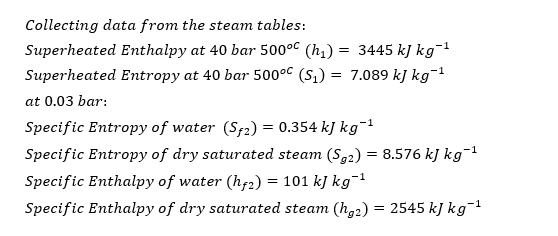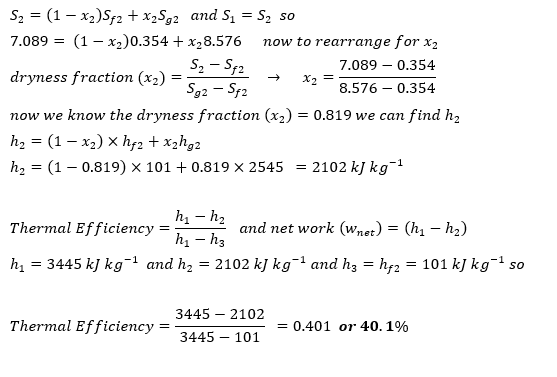#### Attachments

Al_Pa_Cone
This is my next question and below is my attempt so far:

1.Steam at 30 bar and 500°C enters a pass-out turbine at a rate of 18000 kg h–1. The bulk of this steam is expanded isentropically to a condenser pressure of 0.03 bar, but 32% is bled from the turbine at a pressure of 3 bar for process heating. If the condensate from the process heater is directly pumped back to the boiler, find:

(a) the power output from the turbine

First I have collected the data from the steam tables:
h_1 = Enthalpy of superheaded steam = 7.233 kJkg^-1
h_3 = h_f2 = SE of Water at 0.03 bar = 101 kJkg^-1
h_5 = h_f2 = SE of Water at 3 bar = 561 kJkg^-1

for h_2 and h_4 ( the SE of saturated steam ) at 0.03 bar and 3 bar.

As before following my previous method I worked out the dryness fraction first:
for h_4 (the steam bled for process heating at 3 bar) I get:

(S Entropy change in steam at 30bar 500deg) - (S Enthalpy water at 3 bar) / (S Entropy steam at 3 bar) (S Entropy water at 3 bar)

7.233-1.672 / 6.933-1.672 = 1.05 (dryness fraction)

how can the dryness fraction be above 1? and does this mean my previous answer was incorrect?

Any help is much appreciated.

Thanks

Mentor
This just means that the steam at 3 bars is still superheated, and you need to use the superheated steam tables to get its temperature and properties.